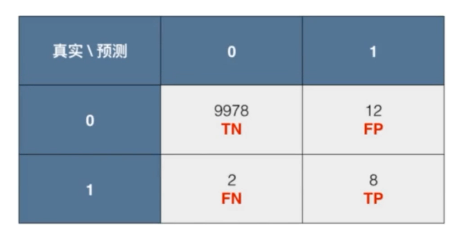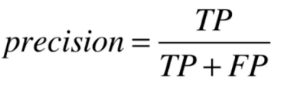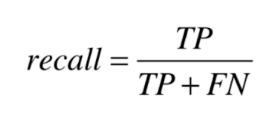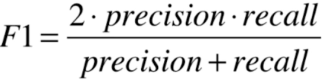• 分类算法评估-混淆矩阵、精确率和召回率 首先引入问题，为什么我们再评估分类算法的时候通常用精确率和召回率? 假设现在有1000个人。预测他们是否得了癌症。假设他们总共有900人是健康的，10人患癌症，那么在这种...
分类算法评估-混淆矩阵、精确率和召回率
首先引入问题，为什么我们再评估分类算法的时候通常用精确率和召回率?
假设现在有1000个人。预测他们是否得了癌症。假设他们总共有900人是健康的，10人患癌症，那么在这种情况下我们如果将所有人都预测为健康的。 在这种情况下我们的准确率是: 99.0% 那我们的这个模型显然的不是一个好的模型。

所以就引出了精确率（precision）和召回率（recall） 先来看一个混淆矩阵这里的T就是True，F就是False，P就是Positive（阳性），N就是Negative（阴性） TN代表的意思也就是：预测的是0 实际也是0 FP代表的意思也就是：预测的是1 实际是0 FN代表的意思也就是：预测的是0 实际是1 TP代表的意思也就是：预测的是1 实际上也是1
然后我们的求精准率的公式就有了 ↓↓↓ 精准率公式：– 召回率公式：文字表达就是**?* 精确率 = 判断为1实际为1 跟 所有预测为1的比例 召回率 = 判断为1实际为1 跟 所有实际为1的比例
这就是精确率和召回率了。其实在不同的情况下所注重的不同，在某些问题上注重精确率，在某些问题上注重召回率。
还有一个评估方法结合了精确率和召回率，那就是F1_Score， F1_Score将精确率和召回率都兼顾了，也就是求了精确率和召回率的调和平均值。
F1_Score的公式为:原			创

展开全文人工智能 机器学习
• 对SVC算法评估结果： 使用ACC评估： Evaluated by accuracy score: [0.95, 0.98, 0.98, 0.98, 0.98, 0.98, 0.97, 0.97, 0.99, 0.97] Average: 0.975 使用F1评估： Evaluated by f1 score: [0.9504950495049505, 0....
产生一组随机的二分类数据，取训练集分别训练NaiveBayes，SVC，随机森林三种算法，对于SVC和随机森林算法，需要额外评估其参数的最佳取值。最后分别使用ACC，f1，rocauc方法评估三种算法。
from sklearn import cross_validation
from sklearn import datasets
from sklearn import naive_bayes
from sklearn import svm
from sklearn import ensemble
from sklearn import metrics
from numpy import argmax

acc_for_NB = []             #使用accuracy评估三个算法
acc_for_SVC = []
acc_for_RFC = []

f1_for_NB = []              # 使用F1-score评估三个算法
f1_for_SVC = []
f1_for_RFC = []

auc_for_NB = []             # 使用AUC ROC评估三个算法
auc_for_SVC = []
auc_for_RFC = []

X, Y = datasets.make_classification(n_samples = 1000, n_features = 10)
kf = cross_validation.KFold(1000, n_folds = 10, shuffle = True)
for train_index, test_index in kf:
X_train, y_train = X[train_index], Y[train_index]
X_test, y_test = X[test_index], Y[test_index]

#使用NB算法
NBclf = naive_bayes.GaussianNB()
NBclf.fit(X_train, y_train)
NBpred = NBclf.predict(X_test)

#分别用三种方法评估NB算法结果
acc_for_NB.append(metrics.accuracy_score(y_test, NBpred))
f1_for_NB.append(metrics.f1_score(y_test, NBpred))
auc_for_NB.append(metrics.roc_auc_score(y_test, NBpred))

#使用SVC算法，先找出最佳的参数C
nn = len(X_train)
Cvalues = [1e-02, 1e-01, 1e00, 1e01, 1e02]
Cscore = []       #记录每个C取值的评估分数
for C in Cvalues:
#将X_train分为5个fold
ikf = cross_validation.KFold(nn, n_folds = 5, shuffle = True)
innerscore = []
#对一个C的取值进行评估
for inner_train_index, inner_test_index in ikf:
inner_X_train, inner_X_test = X_train[inner_train_index], X_train[inner_test_index]
inner_y_train, inner_y_test = y_train[inner_train_index], y_train[inner_test_index]

innerSVCclf = svm.SVC(C = C, kernel = 'rbf')
innerSVCclf.fit(inner_X_train, inner_y_train)
innerSVCpred = innerSVCclf.predict(inner_X_test)
innerscore.append(metrics.accuracy_score(inner_y_test, innerSVCpred))

#记录一个C取值评估分数的平均值，作为该C取值的评估
Cscore.append(sum(innerscore) / len(innerscore))
#取评估结果最好的C取值，进行SVC算法
bestC = Cvalues[argmax(Cscore)]
print("The best C is:", bestC)
SVCclf = svm.SVC(C = bestC, kernel = 'rbf')
SVCclf.fit(X_train, y_train)
SVCpred = SVCclf.predict(X_test)

#用三种方法评估SVC
acc_for_SVC.append(metrics.accuracy_score(y_test, SVCpred))
f1_for_SVC.append(metrics.f1_score(y_test, SVCpred))
auc_for_SVC.append(metrics.roc_auc_score(y_test, SVCpred))

#下面使用RFC算法，先找出最佳n_estimators取值
n_estimators_values = [10, 100, 1000]
n_estimators_scores = []      #记录每个n_estimators评估分数
for n_estimator in n_estimators_values:
ikf = cross_validation.KFold(nn, n_folds = 5, shuffle = True)
innerscore = []
for inner_train_index, inner_test_index in ikf:
inner_X_train, inner_X_test = X_train[inner_train_index], X_train[inner_test_index]
inner_y_train, inner_y_test = y_train[inner_train_index], y_train[inner_test_index]

innerRFCclf = ensemble.RandomForestClassifier(n_estimators=n_estimator)
innerRFCclf.fit(inner_X_train, inner_y_train)
innerRFCpred = innerRFCclf.predict(inner_X_test)
innerscore.append(metrics.accuracy_score(inner_y_test, innerRFCpred))

#记录每个n_estimators评分平均值，作为一个取值的评分
n_estimators_scores.append(sum(innerscore) / len(innerscore))

#取评分最好的n_estimators取值
best_n_estimators = n_estimators_values[argmax(n_estimators_scores)]
print("The best n_estimator is:", best_n_estimators)
RFCclf = ensemble.RandomForestClassifier(n_estimators=best_n_estimators)
RFCclf.fit(X_train, y_train)
RFCpred = RFCclf.predict(X_test)

#用三种方法评估RFC算法
acc_for_RFC.append(metrics.accuracy_score(y_test, RFCpred))
f1_for_RFC.append(metrics.f1_score(y_test, RFCpred))
auc_for_RFC.append(metrics.roc_auc_score(y_test, RFCpred))

print("Naive Bayes:")

print("Evaluated by accuracy score:")
print(acc_for_NB)
print("Average:", sum(acc_for_NB) / len(acc_for_NB))
print()

print("Evaluated by f1 score:")
print(f1_for_NB)
print("Average:", sum(f1_for_NB) / len(f1_for_NB))
print()

print("Evaluated by roc auc score:")
print(auc_for_NB)
print("Average:", sum(auc_for_NB) / len(auc_for_NB))
print()

print("SVC:")

print("Evaluated by accuracy score:")
print(acc_for_SVC)
print("Average:", sum(acc_for_SVC) / len(acc_for_SVC))
print()

print("Evaluated by f1 score:")
print(f1_for_SVC)
print("Average:", sum(f1_for_SVC) / len(f1_for_SVC))
print()

print("Evaluated by roc auc score:")
print(auc_for_SVC)
print("Average:", sum(auc_for_SVC) / len(auc_for_SVC))
print()

print("Random Forest:")

print("Evaluated by accuracy score:")
print(acc_for_RFC)
print("Average:", sum(acc_for_RFC) / len(acc_for_RFC))
print()

print("Evaluated by f1 score:")
print(f1_for_RFC)
print("Average:", sum(f1_for_RFC) / len(f1_for_RFC))
print()

print("Evaluated by roc auc score:")
print(auc_for_RFC)
print("Average:", sum(auc_for_RFC) / len(auc_for_RFC))
print()
将数据集分为10个folds，9个用于训练，1个用于测试。循环作10次测试。每次运行SVC，RFC算法时，对于所有参数可能的取值都要评估，评估方法为将训练数据再分为5个folds，4个用于训练，1个用于测试，取评估分数最高的参数。
The best C is: 1.0
The best n_estimator is: 10
The best C is: 1.0
The best n_estimator is: 1000
The best C is: 1.0
The best n_estimator is: 10
The best C is: 1.0
The best n_estimator is: 10
The best C is: 1.0
The best n_estimator is: 1000
The best C is: 1.0
The best n_estimator is: 100
The best C is: 1.0
The best n_estimator is: 100
The best C is: 1.0
The best n_estimator is: 1000
The best C is: 1.0
The best n_estimator is: 10
The best C is: 1.0
The best n_estimator is: 1000
SVC算法待确定参数为C，RFC算法待确定参数为n_estimator，在10次迭代中，C的最佳取值总是1.0，而n_estimator的三种取值都可能成为最佳取值。
对NaiveBayes算法的评估结果：
使用ACC评估：
Evaluated by accuracy score:
[0.94, 0.96, 0.98, 0.94, 0.94, 0.95, 0.91, 0.95, 0.93, 0.9]
Average: 0.9400000000000001使用F1评估：
Evaluated by f1 score:
[0.9375, 0.9574468085106385, 0.9743589743589743, 0.9189189189189189, 0.9464285714285715, 0.9532710280373831, 0.9072164948453607, 0.9549549549549549, 0.9421487603305785, 0.8979591836734694]
Average: 0.939020369505885使用ROCAUC评估：
Evaluated by roc auc score:
[0.94, 0.9598554797270173, 0.975, 0.9277007145859605, 0.9416564667482661, 0.9487179487179487, 0.909763905562225, 0.9484848484848485, 0.9333616298811546, 0.9007603041216488]
Average: 0.938530129782907

对SVC算法评估结果：
使用ACC评估：
Evaluated by accuracy score:
[0.95, 0.98, 0.98, 0.98, 0.98, 0.98, 0.97, 0.97, 0.99, 0.97]
Average: 0.975使用F1评估：
Evaluated by f1 score:
[0.9504950495049505, 0.9787234042553191, 0.975, 0.9743589743589743, 0.9821428571428572, 0.9807692307692307, 0.968421052631579, 0.972972972972973, 0.991869918699187, 0.9696969696969697]
Average: 0.9744450430032039使用ROCAUC评估：
Evaluated by roc auc score:
[0.95, 0.9799277398635086, 0.9791666666666667, 0.9789827658680118, 0.9824561403508771, 0.9799679487179487, 0.9693877551020409, 0.9686868686868688, 0.9919354838709677, 0.9705882352941176]
Average: 0.9751099604421007

对RFC算法评估结果：
使用ACC评估：
[0.99, 0.97, 0.98, 0.98, 0.98, 0.97, 0.96, 0.96, 0.99, 0.97]
Average: 0.975使用F1评估：
Evaluated by f1 score:
[0.99009900990099, 0.968421052631579, 0.9743589743589743, 0.9743589743589743, 0.9821428571428572, 0.9714285714285713, 0.9583333333333333, 0.9642857142857142, 0.991869918699187, 0.9696969696969697]
Average: 0.9744995375837149使用ROCAUC评估：
Evaluated by roc auc score:
[0.99, 0.9704937775993576, 0.975, 0.9789827658680118, 0.9824561403508771, 0.969551282051282, 0.9595838335334134, 0.9575757575757576, 0.9919354838709677, 0.9705882352941176]
Average: 0.9746167276143785
总结：可以看到SVC和RFC算法在三种方法下的评估结果均比NaiveBayes高，表明SVC和RFC的分类比NaiveBayes更加精确。对于三种评估方法，F1和ROCAUC方法评估结果普遍比ACC高，表明F1与ROCAUC方法的评估标准更加严格。


展开全文• 在以往的分类问题求解当中，我们遇到的问题多为二分类问题，我们常用的评估指标有accuracy, precision, recall_score, f1-score, roc_auc_score等。但是在实际生活中，多分类问题也是大量存在的。这一小节，我们就...
在以往的分类问题求解当中，我们遇到的问题多为二分类问题，我们常用的评估指标有accuracy, precision, recall_score, f1-score, roc_auc_score等。但是在实际生活中，多分类问题也是大量存在的。这一小节，我们就详细的说明一下多分类问题的评估指标。我们先来看一下sklearn库中的二分类的评估指标，以recall_score为例。在recall_score方法中，有一个很重要的参数’average’，它的默认值为’binary’。当在默认参数的情况，该评估方法只能求解二分类问题，如果将该评估方法用于多分类问题，则系统会报错。但’average’同时也向我们提供了其他四个用于解决多分类的问题的参数’micro’,‘macro’,‘weighted’,‘samples’。下面我们以鸢尾花数据集为例来对这四个参数进行逐一说明。 [sklearn.metrics.recall_score]('https://scikit-learn.org/stable/modules/generated/sklearn.metrics.recall_score.html ')
sklearn.metrics.recall_score(y_true, y_pred, labels=None,
pos_label=1, average='binary', sample_weight=None, zero_division='warn')

我们以recall_score的计算为例，recall_score的计算公式如下：

R

e

c

a

l

l

=

T

P

T

P

+

F

N

Recall = \frac {TP}{TP + FN}

为了计算recall_score，我们必须先计算出TP,FN值。我们采用sklearn中的混淆矩阵来计算TP,FN值。
#导入数据分析的常用工具包
import numpy as np
import pandas as pd
import matplotlib.pyplot as plt
import seaborn as sns
%matplotlib inline

#导入iris数据集
from sklearn import datasets

#对iris数据集进行切分
from sklearn.model_selection import train_test_split
X = iris.data
Y = iris.target
X_train,X_test,Y_train,Y_test = train_test_split(X,Y,test_size = 0.3,random_state = 0)

#建立一个基础的逻辑回归模型
from sklearn.linear_model import LogisticRegression
LR = LogisticRegression()
LR.fit(X_train,Y_train)
prediction = LR.predict(X_test)

#计算混淆矩阵
from sklearn.metrics import confusion_matrix
cnf = confusion_matrix(Y_test,prediction,labels = [0,1,2])
print(cnf)
[out]:
array([[16,  0,  0],
[ 0, 13,  5],
[ 0,  0, 11]], dtype=int64)

该分类问题的混淆矩阵如下，列为真实类别，行为预测类别：
class012016001013520011
混淆矩阵（confusion matrix）说明： TP(True positive)：把正例正确地预测为了正例，如把类别0预测为0的个数有16个。 FN(False negative)：把正例错误地预测为了负列，如把类别1预测为2的个数有5个。 FP(False positive)：把负例错误地预测为了正例，假设0为正例，错误地把1,2预测为0就是FP。 TN(True negative)：把负例正确地预测为了负例，假设0为正例，1,2为负例，正确地把1,2预测为1,2就是TN。 对于混淆矩阵，可以这样理解。第一个字母T/F，表示预测的正确与否；第二个字母P/N，表示预测的结果为正例或者负例。如TP就表示预测对了，预测的结果是正例，那它的意思就是把正例预测为了正例。
1.Micro
Micro：把所有类汇总在一起计算出最终recall值，其计算公式如下：

R

e

c

a

l

l

=

T

P

0

+

T

P

1

+

T

P

2

T

P

0

+

F

N

0

+

T

P

1

+

F

N

1

+

T

P

2

+

F

N

2

Recall = \frac{TP_0 + TP_1 + TP_2}{TP_0 + FN_0 + TP_1 + FN_1 + TP_2 + FN_2}

在使用Micro参数时，其recall_score = (16+13+11)/(16+13+11+5) = 0.89。 使用sklearn.metrics方法计算：
from sklearn.metrics import recall_score
recall_score(Y_test,prediction,average = 'micro')
[out]:0.8888888888888888

从上述计算结果可以看出，两者的计算结果是一致的，均为0.89。
2.Macro
Macro：分别计算出每一类的recall值，再取算数平均值，其计算公式如下：

R

e

c

a

l

l

=

(

T

P

0

T

P

0

+

F

N

0

+

T

P

1

T

P

1

+

F

N

1

+

T

P

2

T

P

2

+

F

N

2

)

∗

1

3

Recall =( \frac{TP_0}{TP_0 + FN_0} + \frac{TP_1}{TP_1 + FN_1} + \frac{TP_2}{TP_2 + FN_2}) * \frac{1}{3}

在使用Macro参数时，其recall_score = [16/(16+0+0) + 13/(0+13+5) + 11/(11+0+0)] * 1/3 = 0.91 使用sklearn.metrics方法计算：
from sklearn.metrics import recall_score
recall_score(Y_test,prediction,average = 'macro')
[out]:0.9074074074074074

从上述计算结果可以看出，macro参数下的recall值为0.91。
3.Weighted
Weighted：分别计算每一类的recall值，再乘以各自的权重，然后求和，其计算公式如下：

R

e

c

a

l

l

=

T

P

0

T

P

0

+

F

N

0

∗

W

0

+

T

P

1

T

P

1

+

F

N

1

∗

W

1

+

T

P

2

T

P

2

+

F

N

2

∗

W

2

Recall =\frac{TP_0}{TP_0 + FN_0} * W_0+ \frac{TP_1}{TP_1 + FN_1} * W_1 + \frac{TP_2}{TP_2 + FN_2} * W_2

计算各类的权重：
W0,W1,W2 = np.bincount(Y_test) / len(Y_test)
print(W0,W1,W2)
[out]：0.35555555555555557 0.4 0.24444444444444444

在使用weighted参数时，其recall_score = 16/(16+0+0) * 0.356 + 13/(0+13+5) * 0.4 + 11/(11+0+0) * 0.244 = 0.89 使用sklearn.metrics方法计算：
from sklearn.metrics import recall_score
recall_score(Y_test,prediction,average = 'weighted')
[out]:0.8888888888888888

从上述计算结果可以看出，weighted参数下的recall值为0.89。
4.Samples
samples应用于多标签的分类问题，每一个样本拥有一个以上的标签。如一个感染病毒性肺炎的患者，就可以说他既属于病毒性肺炎患者这一类，也可以说他属于肺炎患者类。
小结： 1.对于多分类算法的评估，我们需要将sklearn.metrics.recall_score中的’average’参数修改为’micro’或’macro’或者’weighted’。 2.在这个例子当中，我们以recall来举例，像其他的评估指标precision, roc_auc_score, f1-score都是采用同样的方法。
文章参考： .https://zhuanlan.zhihu.com/p/59862986
展开全文机器学习 python 数据分析
• mahout中有许多分类器，包括Naive Bayes, Complementary Naive Bayes, Stochastic Gradient ...评估一个分类器（模型）的好坏，需要有一些指标，而在mahout中提供了下列衡量指标： 1. %-correct (ConfusionMatrix
mahout中有许多分类器，包括Naive Bayes, Complementary Naive Bayes, Stochastic Gradient Descent, Support Vector Machine, Random Forest等。评估一个分类器（模型）的好坏，需要有一些指标，而在mahout中提供了下列衡量指标：
1. %-correct  (ConfusionMatrix类)
最简单的，即正确分类的比率
2. Confusion matrix (ConfusionMatrix类)
通常是一个两行两列的矩阵，数据如下：
[ # of true positives, # of false negatives,
# of false positives, # of true negatives]
即为：
[正确分类为正的数量， 错误分类为负的数量，
错误分类为正的数量， 正确分类为负的数量]
一个较好的模型，应该是true positives和true negatives都远大于false negatives和false positives。
注意：false negative的代价实际上比false positive高得多。举例来说，垃圾邮件分类。正确分类为垃圾邮件为true positive，则将垃圾邮件分类为普通邮件（相当于未能将垃圾邮件正确分类为垃圾邮件）为false positive，将普通邮件分类为垃圾邮件为false negative。显然，将普通邮件分类成垃圾邮件的代价是远高于将垃圾邮件分类为普通邮件的。
confision matrix也可能是包含了所有分类结果的：
Confusion Matrix ------------------------------------------------------- a   b   c   d   e   f  <--Classified as 9   0   1   0   0   0  |  10  a = one 0   9   0   0   1   0  |  10  b = two 0   0   10 0   0   0  |  10  c = three 0   0   1   8   1   0  |  10  d = four 1   1   0   0   7   1  |  10  e = five 0   0   0   0   1   9  |  10  f  = six Default Category: one: 6
3. Entropy matrix (Auc类)
跟confusion matrix类似，但把具体的数量值变成了概率对数平均值。因而跟confusion matrix刚好相反，一个好的模型应该具有较小的对角线值。
4. AUC (Area under curve) (Auc, OnlineAuc, CrossFoldLearner, AdaptiveLogisticRegression)
简单地说，就是能够正确分类的概率。若AUC=0，则完全不能正确分类，模型很差；若AUC=1，则是个完美的模型。AUC只能用于二分类。

展开全文matrix vector random c
• ## 机器学习之KNN最邻近分类算法

万次阅读 多人点赞 2018-09-15 13:13:33
KNN（K-Nearest Neighbor）最邻近分类算法是数据挖掘分类（classification）技术中最简单的算法之一，其指导思想是”近朱者赤，近墨者黑“，即由你的邻居来推断出你的类别。 KNN最邻近分类算法的实现原理：为了...KNN python
•   要知道算法模型对未知数据表现如何，最好的评估办法是利用已经明确知道结果的数据运行生成的算法模型进行验证。此外，还可以采用重新采样评估的方法，使用新的数据来评估算法模型。   1.评估算法的方法   ...
• 混淆矩阵针对二分类问题，将实例分成正类(postive)或者负类(negative)。但是实际中分类时，会出现四种情况. (1)若一个实例是正类并且被预测为正类，即为真正类(True Postive TP) (2)若一个实例是正类，但是被预测...数据挖掘 Python
• 关于分类算法我们之前也讨论过了KNN、决策树、naivebayes、SVM、ANN、logistic回归。关于这么多的分类算法，我们自然需要考虑谁的表现更加的优秀。  既然要对分类算法进行评价，那么我们自然得有评价依据。到目前...
• 基于决策树的启发式流分类算法目标是建立结点数目尽可能少、树深度尽可能小的数据结构，从而获得较优的时空性能，据此理论提出的基于参数评估的可调节式流分类算法（PEA），一方面沿袭目前主流的决策树类流分类算法...
• 其实，回归算法中也有类似分类算法，回归算法在机器学习中就是为了解决分类问题。 至于这个分类模型有什么用，我们在机器学习过程中： 定义一个对象X，将其划分到定义的某个类别Y中，输出是某个类别，例如新闻类，...NB 朴素贝叶斯
• 内容提要数据集英文语料中文语料评估指标召回率与准确率F1-评测值微平均与宏平均混淆矩阵   优秀的文本分类模型必须经得住真实数据集的验证，因而分类器必须在通用的数据集上进行测试。用于文本分类的数据集一般...自然语言处理 机器学习
• 分类不平衡协议流的机器学习算法评估与比较.pdf
• 该项目的目标是评估应用于流数据的9种分类算法的准确性。 首先，创建了一个实时数据管道，该管道： 用RandomRBF生成器创建数据源 使用Apache Flink将数据拆分为80％的训练和20％的测试集 附加了Massive Online ...
• 大部分文本分类系统包含特征抽取、降维、... 分类器：分类算法 评估：预测测试集和评估模型，F Score、ROC以及AUC等 目前采用的几种特征抽取方法没有考虑句法结构信息，例如下图中的指示代词 CNN HAN ...
• 1.什么是分类算法 2.关于分类算法的问题 3.决策树算法介绍 4.评估模型的准确性 5.应用：决策树算法在python中的实现 6.总结
• ## 目标检测算法评估

千次阅读 2019-04-08 22:23:48
目标检测算法评估 目录 目标检测的评估 引论 precision和recall PR曲线和AP，mAP VOC的mAP评价代码 总结 引论 现在关于深度学习的算法层出不穷，那么我们应该用什么指标去评判一个算法的优缺点呢？ 以...
• 决策公平 该存储库包含用于评估分类算法上的公平性指标的代码。
•研究论文
• 时间序列分类算法A common task for time series machine learning is classification. Given a set of time series with class labels, can we train a model to accurately predict the class of new time series?...python java 人工智能 机器学习
• ## 分类算法评价

千次阅读 2017-04-26 21:33:56
不同的分类算法有不同的特征，在不同的数据集上表现的效果也不同，我们需要根据特定的任务进行算法的选择，如何选择分类，如何评价一个分类算法的好坏，前面关于决策树的介绍，我们主要用的正确率（accuracy）来评价...
• 不同的分类算法有不同的特定，在不同的数据集上表现的效果也不同，我们需要根据特定的任务进行算法的选择，如何选择分类，如何评价一个分类算法的好坏，前面关于决策树的介绍，我们主要用的正确率（accuracy）来评价...
• ## 分类算法评价标准

千次阅读 2017-04-06 16:37:08
一、引言分类算法有很多，不同分分类算法又用很多不同的变种。不同的分类算法有不同的特定，在不同的数据集上表现的效果也不同，我们需要根据特定的任务进行算法的选择，如何选择分类，如何评价一个分类算法的好坏，...机器学习 文本处理
• ## 分类算法简述

万次阅读 多人点赞 2016-05-21 16:56:32
分类算法简述一、什么是分类算法数据挖掘任务通常分为两大类： 预测任务，根据其他属性的值，预测特定属性的值。 描述任务，概括数据中潜在联系的模式（相关性，趋势，聚类，轨迹和异常） 　分类属于预测任务，...数据挖掘
• 了解分类算法评估标准准确率 应用：Facebook签到位置预测 1 K-近邻算法(KNN) 1.1 定义 如果一个样本在特征空间中的k个最相似(即特征空间中最邻近)的样本中的大多数属于某一个类别，则该样本也属于这个类别，即...K-近邻算法 特征工程处理
• 第一篇：从决策树学习谈到贝叶斯分类算法、EM、HMM （Machine Learning & Data Mining交流群：8986884）引言 最近在面试中，除了基础 & 算法 & 项目之外，经常被问到或被要求介绍和描述下自己所知道...vector 自然语言处理 string 数据挖掘
• 针对传统分类算法在处理短文本时的不足，提出了一种基于搜索的NaiveBayes文本分类方法。...并且在分类算法、分类器构造和评估3方面进行了详细的介绍。实验证明，基于搜索的文本分类器对于短文本有更好的分类效果。
• 分类算法这一块，本篇项目总共使用了 《14》 种分类算法来进行文本分类， 涵盖《sklearn》中的常规分类算法和集成学习算法; 竞赛和工业界比较得宠的集成学习算法《xgboost》和《lightgbm》; 深度学习框架《Kerpython 深度学习 人工智能 机器学习
• ## K近邻分类算法

千次阅读 2018-09-19 15:59:25
K最近邻(k-Nearest Neighbor，KNN)分类算法，是一个理论上比较成熟的方法，也是最简单的机器学习算法之一。该方法的思路是：如果一个样本在特征空间中的k个最相似(即特征空间中最邻近)的样本中的大多数属于某一个...AI...